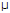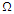# Online Digital Electronics Test - Digital Electronics Test 10Loading Test...

Instruction:

• This is a FREE online test. DO NOT pay money to anyone to attend this test.
• Total number of questions : 20.
• Time alloted : 30 minutes.
• Each question carry 1 mark, no negative marks.
• DO NOT refresh the page.
• All the best :-).

1.

If a typical PC uses a 20-bit address code, how much memory can the CPU address?

A.
 20 MBB.
 10 MBC.
 1 MBD.
 580 MB2.

Fan-out is specified in terms of ________.

A.
 voltageB.
 currentC.
 wattageD.
 unit loads3.

Which of the following is true for a 5-variable Karnaugh map?

A.
 There is no such thing.B.
 It can be used only with the aid of a computer.C.
 It is made up of two 4-variable Karnaugh maps.D.
 It is made up of a 2-variable and a 3-variable Karnaugh map.4.

One reason for using the sum-of-products form is that it can be implemented using all ________ gates without much difficulty.

A.
 NORB.
 NANDC.
 ANDD.
 DOOR5.

A D flip-flop utilizing a PGT clock is in the CLEAR state. Which of the following input actions will cause it to change states?

A.
 CLK = NGT, D = 0B.
 CLK = PGT, D = 0C.
 CLOCK NGT, D = 1D.
 CLOCK PGT, D = 1E.
 CLK = NGT, D = 0, CLOCK NGT, D = 16.

The duty cycle of the most significant bit from a 4-bit (0–9) BCD counter is ________.

A.
 10%B.
 20%C.
 50%D.
 80%7.

To which pin on the RAM chip does the address decoder connect in order to signal which memory chip is being accessed?

A.
 The address inputB.
 The output enableC.
 The chip enableD.
 The data input8.

What is another name for digital circuitry called sequential logic?

A.
 logic macrocellB.
 logic arrayC.
 flip-flop memory circuitryD.
 inverter9.

A GAL is a programmable/reprogrammable PAL.

A.
 TrueB.
 False10.

Which type of programming is typically used for digital signal processors?

A.
 Assembly languageB.
 Machine languageC.
 CD.
 None of the above11.

An offset error in a DAC will show up as an incorrect analog output ________.

A.
 only for higher value inputsB.
 only for lower value inputsC.
 only for certain (scattered) inputsD.
 for all inputs12.

A certain gate draws 1.8A when its output is HIGH and 3.3 µA when its output is LOW. VCC is 5 V and the gate is operated on a 50% duty cycle. What is the average power dissipation (PD)?

A.
 2.55WB.
 1.27WC.
 12.75WD.
 5W13.

CMOS is a more dominant IC technology than TTL.

A.
 TrueB.
 False14.

A NAND gate has:

A.
 active-LOW inputs and an active-HIGH output.B.
 active-LOW inputs and an active-LOW output.C.
 active-HIGH inputs and an active-HIGH output.D.
 active-HIGH inputs and an active-LOW output.15.

The 2's-complement system is to be used to add the signed binary numbers 11110010 and 11110011. Determine, in decimal, the sign and value of each number and their sum.

A.
 –113 and –114, –227B.
 –14 and –13, –27C.
 –11 and –16, –27D.
 –27 and –13, –4016.

CMOS stands for "complementary metal-oxide semiconductors" and the FETs are normally enhancement mode devices.

A.
 TrueB.
 False17.

In an HDL application of a stepper motor, after an up/down counter is built what is done next?

A.
 Build the sequencerB.
 Test it on a simulatorC.
 Test the decoderD.
 Design an intermediate integer variable18.

On a binary-weighted D/A converter the least significant binary input ________.

A.
 connects to the smallest resistorB.
 supplies the least voltageC.
 connects to a 1 kresistorD.
 connects to the largest resistor19.

When two or more inputs are active simultaneously, the process is called:

A.
 first-in, first-out processingB.
 priority encodingC.
 ripple blankingD.
 first-in, first-out processing or priority encoding20.

The number of data registers is:

A.
 8B.
 16C.
 32D.
 64